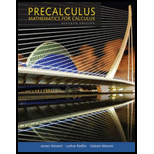# The polar equation in rectangular coordinates. Graph this equation in circle form and find the radius and center of the circle.### Precalculus: Mathematics for Calcu...

7th Edition
James Stewart + 2 others
Publisher: Brooks Cole
ISBN: 9781305071759### Precalculus: Mathematics for Calcu...

7th Edition
James Stewart + 2 others
Publisher: Brooks Cole
ISBN: 9781305071759

#### Solutions

Chapter 8.2, Problem 61E

(a)

To determine

## To evaluate: The polar equation in rectangular coordinates. Graph this equation in circle form and find the radius and center of the circle.

(b)

To determine

### Want to see the full answer?

Check out a sample textbook solution.

### Want to see this answer and more?

Experts are waiting 24/7 to provide step-by-step solutions in as fast as 30 minutes!*

*Response times may vary by subject and question complexity. Median response time is 34 minutes for paid subscribers and may be longer for promotional offers.